• 今天小编就为大家分享一篇python实现逆滤波与维纳滤波示例，具有很好的参考价值，希望对大家有所帮助。一起跟随小编过来看看吧
• 维纳滤波matlab代码，对语音去噪有较明显效果，可做去噪算法借鉴
•维纳滤波
• 图像去模糊，各种传统滤波算法，逆滤波、维纳滤波、LR算法等等。。。针对图像运动模糊领域
• 图像处理课程作业 可直接运行 附带评价...通过维纳滤波实现图像复原。并与逆滤波的方法进行对比。最后采用PSNR和MSE对维纳滤波的结果进行评价。 由于存在取整误差，就算去掉高斯噪声，逆滤波仍然难以完全还原原始图像。
• 1. 加性高斯白噪声1.1 特点加性高斯白噪声属于白噪声的一种，有如下两个特点：功率谱密度为常数，即白高斯则为其幅度的概率密度函数服从高斯分布1.2 高斯分布的一维概率密度函数 为标准差为均值1.3 Python内置库说明...

1. 加性高斯白噪声

1.1 特点

加性高斯白噪声属于白噪声的一种，有如下两个特点：

功率谱密度为常数，即白

高斯则为其幅度的概率密度函数服从高斯分布

1.2 高斯分布的一维概率密度函数为标准差为均值

1.3 Python内置库说明

random.gauss(mu, sigma)其值即服从高斯分布，若想要是实现加性高斯白噪声，循环作加即可

1.4 Code

import numpy as np

import random

def gaussian_white_noise(intput_signal, mu, sigma):

'''

加性高斯白噪声（适用于灰度图）

:param intput_signal: 输入图像

:param mu: 均值

:param sigma: 标准差

:return:

'''

intput_signal_cp = np.copy(intput_signal) # 输入图像的副本

m, n = intput_signal_cp.shape # 输入图像尺寸（行、列）

# 添加高斯白噪声

for i in range(m):

for j in range(n):

intput_signal_cp[i, j] = intput_signal_cp[i, j] + random.gauss(mu, sigma)

return intput_signal_cp

1.5 测试对比均值为0，标准差为10时的加行高斯白噪声前后对比图

2. 逆滤波

实际上逆滤波是维纳滤波的一种理想情况，当不存在加性噪声时，维纳滤波与逆滤波等同。

2.1 基本原理

在时域内有为输入信号为系统函数为输出信号

根据时域卷积定理，我们知道时域卷积等于频域乘积分别为输出信号，输入信号，系统函数的傅里叶变换

则有这意味着，当我们已知系统函数时，我们可以很简单的完成滤波。

2.2 Code

import numpy as np

def inverse_filtering(input_singal, h):

'''

逆滤波

:param input_singal: 输入信号

:param h: 退化函数（时域）

:return: 滤波后的信号（幅值）

'''

output_signal = [] #输出信号

output_signal_fft = [] #输出信号的傅里叶变换

h_fft = np.fft.fft2(h) # 退化函数的傅里叶变换

input_singal_fft = np.fft.fft2(input_singal) # 输入信号的傅里叶变换

output_signal_fft = input_singal_fft / h_fft

output_signal = np.abs(np.fft.ifft2(output_signal_fft))

return output_signal

3 维纳滤波

3.1 直观认识

理解了逆滤波的基本过程之后，实际上维纳滤波就不是太大问题了。实际上，逆滤波对于绝大多数情况滤波效果都不好，因为逆滤波是通过傅里叶变换将信号由时域转换到频域，再根据时域卷积定理，在频域作除法。对于乘性干扰这当然是没问题的，甚至是完美的。而如果存在加性噪声，例如：加性高斯白噪声。逆滤波效果就不好了，某些情况下几乎无法完成滤波情况。

3.2 数学解释

输入信号经过系统函数后

时域上频域上若存在加性噪声则为

时域上其中频域上其中于是，从上面对输入信号的估计表达式可以看出，多出了一项加性噪声的傅里叶变换与系统函数的比值。尤其当相对于很小时，滤波后的信号差距十分严重。

3.3 维纳滤波表达式而我们又知道：白噪声的白为噪声的功率谱为常数，即为常数，于是，从直观上看，当相对于较大时，则较小，上式第一项则较小，而第二项较大从而保持相对平稳。

3.4 Code

import numpy as np

def wiener_filtering(input_signal, h, K):

'''

维纳滤波

:param input_signal: 输入信号

:param h: 退化函数（时域）

:param K: 参数K

:return: 维纳滤波后的信号（幅值）

'''

output_signal = [] # 输出信号

output_signal_fft = [] # 输出信号的傅里叶变换

input_signal_cp = np.copy(input_signal) # 输入信号的副本

input_signal_cp_fft = np.fft.fft2(input_signal_cp) # 输入信号的傅里叶变换

h_fft = np.fft.fft2(h) # 退化函数的傅里叶变换

h_abs_square = np.abs(h_fft)**2 # 退化函数模值的平方

# 维纳滤波

output_signal_fft = np.conj(h_fft) / (h_abs_square + K)

output_signal = np.abs(np.fft.ifft2(output_signal_fft * input_signal_cp_fft)) # 输出信号傅里叶反变换

return output_signal

4 维纳滤波与逆滤波对比cameracoins

5 GitHub

展开全文• python 实现小波维纳滤波

千次阅读 2019-12-15 10:11:05
通过在小波域高频处，进行维纳滤波，来降低噪声对图像的影响。（假设噪声分布呈独立同分布） import os import cv2 from matplotlib import pyplot as plt import numpy as np import pywt # print(pywt....
通过在小波域高频处，进行维纳滤波，来降低噪声对图像的影响。（假设噪声分布呈独立同分布）
import os
import cv2
from matplotlib import pyplot as plt
import numpy as np
import pywt

# print(pywt.families())
# ['haar', 'db', 'sym', 'coif', 'bior', 'rbio', 'dmey', 'gaus', 'mexh', 'morl', 'cgau', 'shan', 'fbsp', 'cmor']

def guass_noise(pic, SNR = 1):
#SNR为信噪比
pic = np.array(pic, dtype = float)
SNR = 10 ** (SNR / 10)
row, col = np.shape(pic)
pic_power = np.sum(pic * pic) / (row * col)
noise_power = pic_power / SNR
noise = np.random.randn(row, col) * np.sqrt(noise_power)
pic = (noise + pic)
pic = np.where(pic <= 0, 0, pic)
pic = np.where(pic >255, 255, pic)
return np.uint8(pic)

def SNF_calcu(pic, pic_):
#pic 原图  pic_被污染的图片
pic = np.array(pic, dtype = float)
pic_ = np.array(pic_, dtype=float)
noise = pic - pic_
return 10 * np.log10(np.sum(pic * pic) / np.sum(noise * noise))

def wiener_filter(pic, HH):
row, col = np.shape(pic)
noise_std = (np.median(np.abs(HH)) / 0.6745)
noise_var = noise_std ** 2
var = 1 / (row * col) * np.sum(pic * pic) - noise_var
ans = pic * var / (var + noise_var)
return ans

def wiener_dwt(pic, index = 1):
#index 为进行几层分解与重构
pic = np.array(pic, dtype = float)
coeffs = pywt.dwt2(pic, 'bior4.4')
LL, (LH, HL, HH) = coeffs

#LL为低频信号 LH为水平高频 HL为垂直高频  HH为对角线高频信号

#维纳滤波
LH = wiener_filter(LH, HH)
HL = wiener_filter(HL, HH)
HH = wiener_filter(HH, HH)

#重构
if index > 1:
LL = wiener_dwt(LL, index - 1)
#bior4.4小波重构可能会改变矩阵维数，现统一矩阵维数
row, col = np.shape(LL)
d1 = row - np.shape(HH)
d2 = col - np.shape(HH)
if d1 > 0 or d2 > 0:
d1 = row - np.arange(d1) - 1
d2 = col - np.arange(d2) - 1
LL = np.delete(LL, d1, axis = 0)
LL = np.delete(LL, d2, axis = 1)
plt.figure()
plt.subplot(2, 2, 1)
plt.imshow(LL, cmap='gray')
plt.title('The ' + str(time - index + 1) + 'th dwt----' + 'LL')
plt.subplot(2, 2, 2)
plt.imshow(LH, cmap='gray')
plt.title('The ' + str(time - index + 1) + 'th dwt----' + 'LH')
plt.subplot(2, 2, 3)
plt.imshow(HL, cmap='gray')
plt.title('The ' + str(time - index + 1) + 'th dwt----' + 'HL')
plt.subplot(2, 2, 4)
plt.imshow(HH, cmap='gray')
plt.title('The ' + str(time - index + 1) + 'th dwt----' + 'HH')
plt.show()
pic_ans = pywt.idwt2((LL, (LH, HL, HH)), 'bior4.4')
# pic_ans = np.where(pic_ans <= 0, 0, pic_ans)
# pic_ans = np.where(pic_ans > 255, 255, pic_ans)
return pic_ans

def run(filename):
SNR = 22
global time
time = 5    #分解次数
f_noise = guass_noise(f, SNR)
f_process = wiener_dwt(f_noise, time)
f_process = np.where(f_process <= 0, 0, f_process)
f_process = np.where(f_process > 255, 255, f_process)
f_process = np.uint8(f_process)

print(SNF_calcu(f, f_noise))
print(SNF_calcu(f, f_process))
plt.figure()
plt.subplot(1, 3, 1)
plt.imshow(f, cmap = 'gray')
plt.title('original image')
plt.subplot(1, 3, 2)
plt.imshow(f_noise,cmap = 'gray')
plt.title('polluted image ----SNR = ' + str(SNR))
plt.subplot(1, 3, 3)
plt.imshow(f_process, cmap='gray')
plt.title('polluted image after wiener_dwt')
plt.show()

if __name__ == "__main__":
run('lena.tiff')

最终效果图：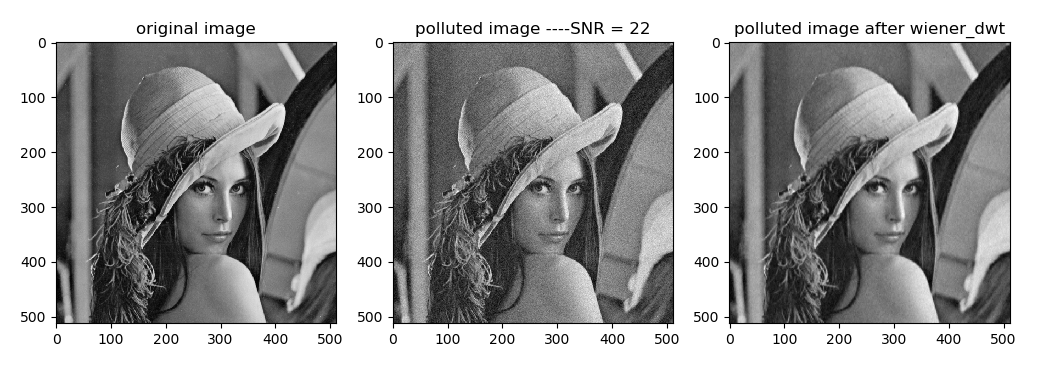原图信噪比与处理后图片信噪比：

SNF of polluted image is 21.985682296401862
SNF of processed image is 25.442700349930245

展开全文滤波 python 小波变换
• 维纳滤波

万次阅读 多人点赞 2016-12-17 16:55:43
之前所接触的低通/带通/高通滤波器和匹配滤波器等...维纳滤波原理维纳滤波器通常用于提取被噪声污染的有用信号，它是以最小均方误差准则进行滤波的，下面对该准则下的最优滤波器系数进行推导。 图1.1 维纳滤波器

之前所接触的低通/带通/高通滤波器和匹配滤波器等，都是应用于要求“最佳”系数不随时间变化的场合。但是，在通信、雷达、语音处理和生物医学等领域，通常需要滤波器系数能够根据输入信号进行自适应的调整。本文主要介绍FIR方式实现的维纳滤波器。

维纳滤波原理

维纳滤波器通常用于提取被噪声污染的有用信号，它是以最小均方误差准则进行滤波的，下面对该准则下的最优滤波器系数进行推导。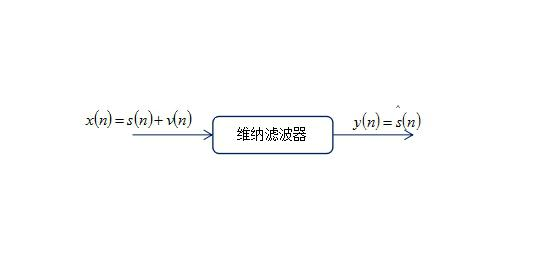图1.1 维纳滤波器


如图1.1所示，输入 x(n) $x(n)$ s(n) $s(n)$为有用信号， v(n) $v(n)$为噪声干扰。输出 y(n) $y(n)$为对有用信号 s(n) $s(n)$的估计 s(n) $s ^{\wedge} (n)$
s(n) $s(n)$是我们期望得到的信号，称之为期望信号。 s(n) $s ^{\wedge} (n)$为滤波器实际输出的观测信号。那么误差定义应为：
e=ss

均方误差为:
J=E(e2)

我们的目的就是要推导使 J $J$达到最小时的滤波器系数。
J=E{(ss)2}  =E{(swHx)2}=E{s22swHx+wHxxHw}

其中 w $w$表示滤波器系数。
从上面式子可以看出， 代价函数/成本函数J$J$ w $w$的一个二次函数，在以w$w$为坐标轴的坐标系中是一个凹函数，有唯一的一个极值点，只要求出该极值点，就可以得到使代价函数 J $J$最小的滤波器权值w$w$
J $J$w$w$求偏导：
=JwH=E{2sx+2xHxw}

令其为 0 $0$，即可得到极值点：
wopt={E(xxH)}1E(dx)

其中 E(xxH) $E(xx^H)$为输入信号的自相关， E(dx) $E(dx)$为期望信号与输入信号的互相关。
通常写成更简洁的矩阵形式：
wopt=R1xxRdx

由于实际中无法得到输入信号的理想统计参数，通常利用实际输入信号的采样来进行自相关系数矩阵和互相关系数矩阵的无偏估计。
该公式也称为维纳-霍夫方程，是最小均方误差准则下的滤波器系数最优解。

滤波器应用

维纳滤波器的应用十分广泛，总体上可以分为下面四类。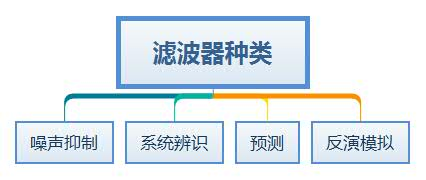图2.1 维纳滤波器


下面分别对这四种情况进行介绍和仿真。

噪声抑制

这种应用较为常见，就是从噪声中提取有用信号，结构图如下：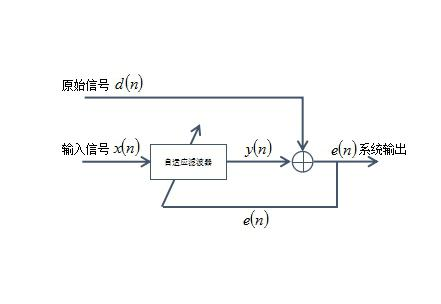图2.2 噪声抑制


如图所示，原始信号 d(n) $d(n)$就是包含了噪声干扰的有用信号，而输入信号 x(n) $x(n)$是与 d(n) $d(n)$中的噪声干扰相关的一个基准信号，通过维纳滤波器，利用基准信号 x(n) $x(n)$ d(n) $d(n)$中的噪声干扰进行逼近，最后用原始信号减去滤波器输出即可得到估计的有用信号。
这里用电力线信号的传输作为例子，假设电力线中传递了一个编码信号，而除去信道噪声外，通常还有一个交流电 50Hz $50Hz$的干扰，这时候就有必要对交流电干扰进行消除，下面是对该情形的仿真。
信道传输的信道编码信号如下所示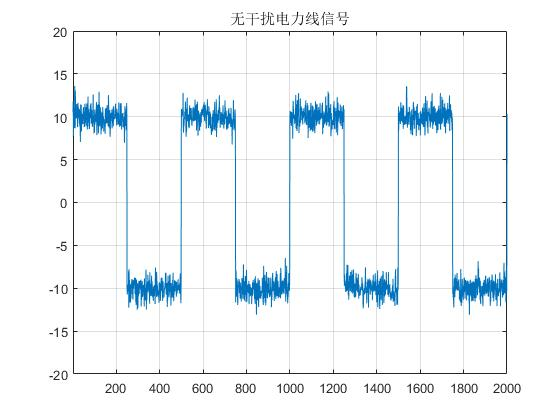图2.3 电力线编码信号


添加 50Hz $50Hz$交流电干扰信号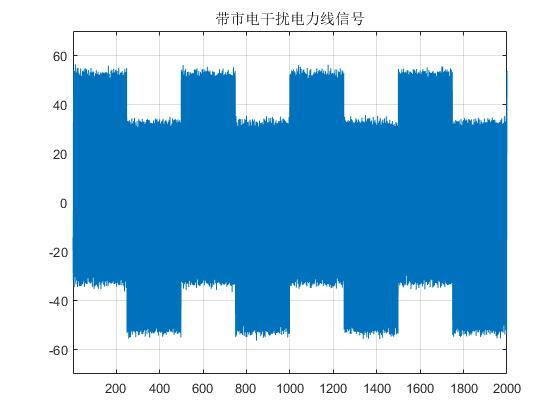图2.4 带交流电干扰电力线编码信号


接下来采用一个两阶的维纳滤波器，进行自相关和互相关矩阵的计算，并进行滤波。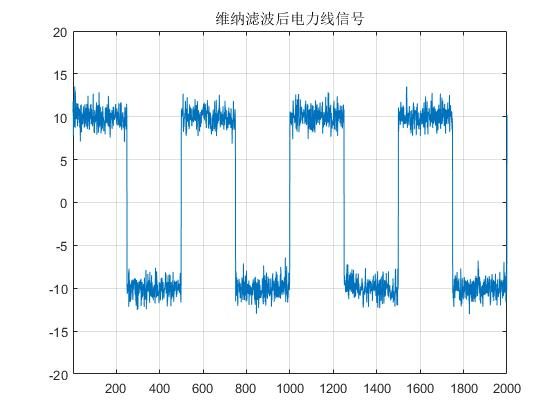图2.5 滤波后的电力线信号


如图2.5所示，在仿真情况下信号得到了比较好的恢复。

代码附录

clc ; clear ; close all;

T = 10;    % 取10s信号进行分析
f0 = 50;   % 市电干扰频率
fs = 4*f0; % 采样频率

% generate observed signal
for k = 1:1:T*fs
if mod(floor(k/(T*fs/8)),2) == 0
d(k) = 10;
else
d(k) = -10;
end
end
d = d + normrnd(0,1,1,T*fs); % add noise

figure; plot(d); title('无干扰电力线信号'); grid on; axis([1 T*fs -20 20])

n = 1:1:T*fs;
d = d + 50*cos(2*pi*f0*n/fs + pi/6); % pi/6 --- phase of interference

figure; plot(d); title('带市电干扰电力线信号');grid on; axis([1 T*fs -70 70])

% generate reference signal   order = 1  /  coeff number = 2
x_in1 = cos(2*pi*f0*n/fs);
x_in2 = cos(2*pi*f0*(n-1)/fs);
x = [x_in1;x_in2];

% wiener filter
R_xx = x*x'/(T*fs);     % E(xx')
R_dx = d*x'/(T*fs);     % E(dx')
w = R_xx\(R_dx.');
y = d - w'*x;

figure; plot(y); title('维纳滤波后电力线信号');grid on; axis([1 T*fs -20 20])

系统辨识

在系统辨识应用中，维纳滤波通常来确定未知系统的滤波器系数。系统辨识的结构如图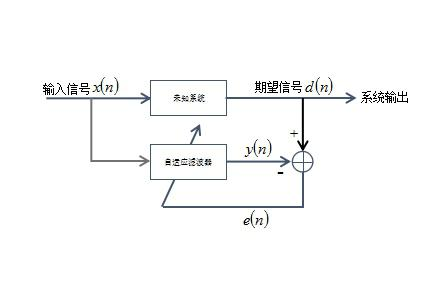图2.6 系统辨识结构图


当然，在实际系统中，系统输出会带有信道噪声，自适应滤波器结构无法与未知系统完全匹配。
下面进行一个简单的仿真，假设输入信号 x(n) $x(n)$为高斯白噪声，通过的未知系统为低通滤波器，利用滤波器输出 d(n) $d(n)$和系统输入 x(n) $x(n)$来对未知系统进行估计。
产生的高斯白噪声 x(n) $x(n)$频谱：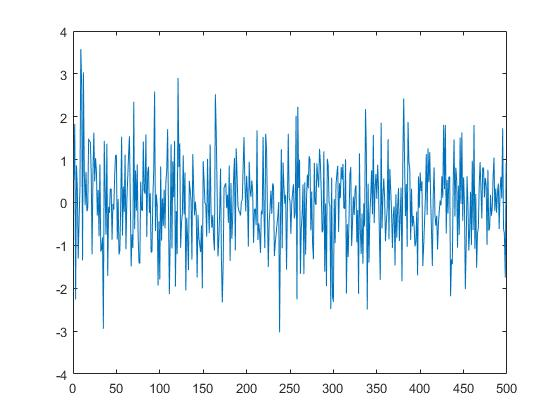图2.7 输入的高斯白噪声频谱


将该噪声通过未知系统（低通滤波器），得到的系统输出 d(n) $d(n)$频谱为：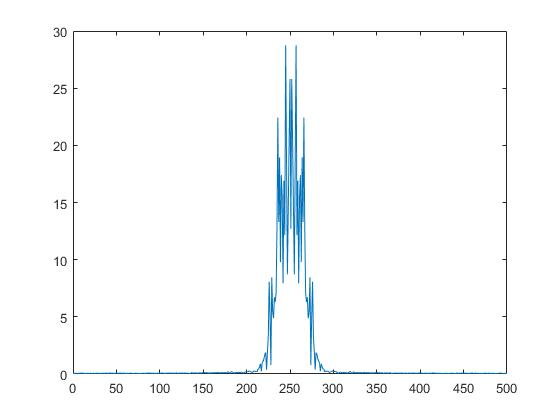图2.8 系统输出的频谱


利用上面两个信号，构建维纳滤波器，得到维纳滤波器的系数幅频/相频特性为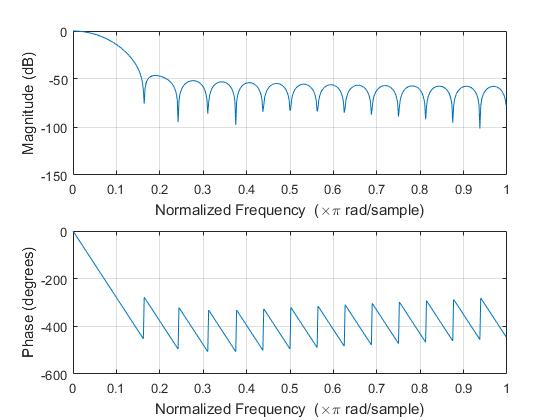图2.9 维纳滤波器幅频/相频特性


下面给出未知系统的幅频/相频特性，可以看出两者基本是一致的。图2.9 未知系统幅频/相频特性


代码附录

% 系统辨识
clc ; clear ; close all;

x = normrnd(0,1,1,500); % input x(n)
b = fir1(31,0.05);      % fir filter coeff
d = filter(b,1,x);      % output d(n)

figure;    freqz(b);    title('未知系统幅频/相频特性')
figure;    plot(abs(fftshift(fft(x))));    title('输入信号x(n)')
figure;    plot(abs(fftshift(fft(d))));    title('未知系统输出d(n)')

x_in = zeros(32,500); % delay  0 to 31    filter order = 31
for k = 1:1:32
x_in(k,:) = [zeros(1,k-1)  x(1:500-k+1)];
end

R_xx = x_in*x_in'/500;
R_dx = d*x_in'/500;
w = inv(R_xx)*R_dx.';
y = w'*x_in;

figure;    plot(abs(fftshift(fft(y))));  title('维纳滤波器输出信号频谱')
figure;    freqz(w);    title('维纳滤波器幅频/相频特性')

反演模拟

在反演模拟中，自适应滤波器的任务是提供表示未知的时变被控对象的最佳拟合的反演模型。它可用于滤波器的设计等场景中。具体结构如下图所示。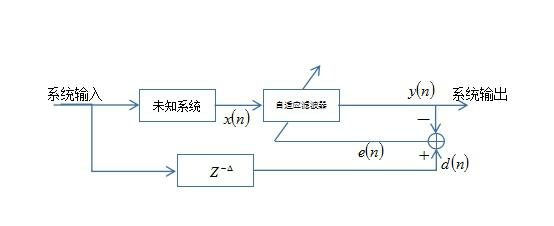图2.10 反演模拟结构


输入信号 d(n) $d(n)$进入未知系统，得到维纳滤波器的输入 x(n) $x(n)$,而延迟后的输入信号 d(n) $d(n)$2用于计算误差信号 e(n) $e(n)$，最后得到的维纳滤波器的权值就是对未知系统的反演。
下面假设输入信号 d(n) $d(n)$是高斯白噪声，未知系统为低通滤波器，未知系统的幅频/相频特性和得到的维纳滤波器的系数幅频/相频特性分别为。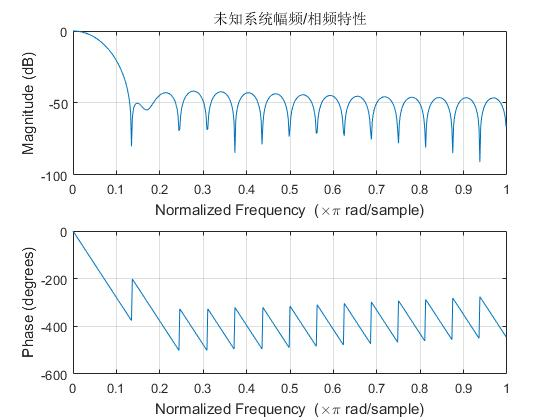图2.11 低通滤波器结构


得到的维纳滤波器的幅频/相频特性为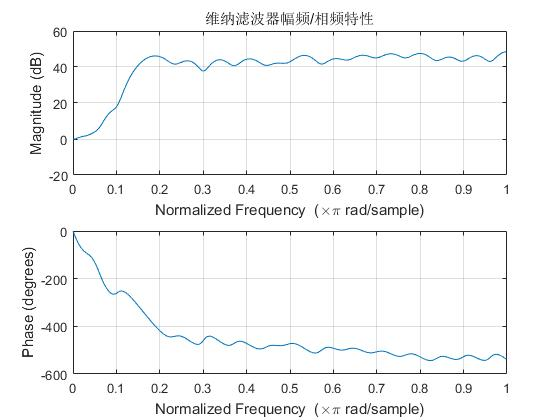图2.12 维纳滤波器结构



代码附录

clc ; clear ; close all;

d = normrnd(0,1,1,500);     % input d(n)
b = fir1(31,0.001);         % fir filter coeff
x = conv(d,b);              % output x(n)
figure;    freqz(b);    title('未知系统幅频/相频特性')
figure;    plot(abs(fftshift(fft(d))));    title('输入信号d(n)')
figure;    plot(abs(fftshift(fft(x))));    title('未知系统输出x(n)')
x_in = zeros(32,500); % delay  0 to 31    filter order = 31
for k = 1:1:32
x_in(k,:) = [zeros(1,k-1) x(1:500-k+1) ];
end
d = [zeros(1,32) d(1:500-32) ]; % delay
R_xx = x_in*x_in'/500;
R_dx = d*x_in'/500;
w = inv(R_xx)*R_dx.';
y = w'*x_in;
figure;    plot(abs(fftshift(fft(y))));  title('维纳滤波器输出信号频谱')
figure;    fritle('维纳滤波器幅频/相频特性')

预测

当输入信号与白噪声有本质区别时，可将维纳滤波器作为预测器使用，结构如下图所示。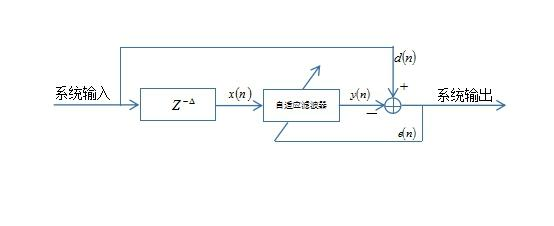图2.13 预测器结构


下面用一个带高斯白噪声的正弦信号作为系统输入，用于实验维纳滤波的估计效果。
输入信号如下图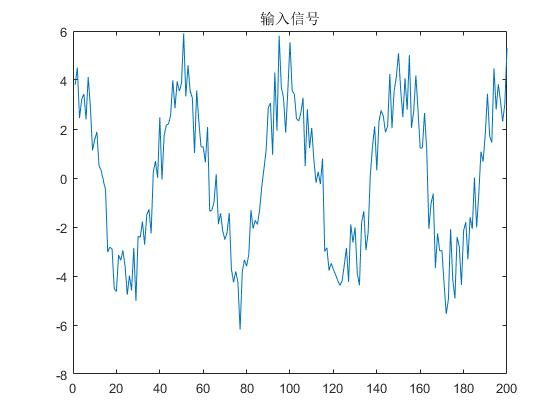图2.13 预测器输入


预测器输出与输入对比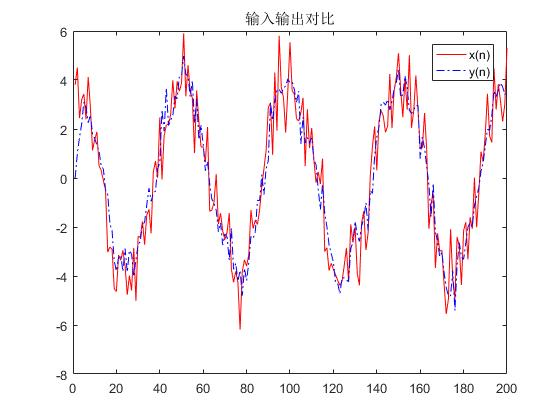图2.14 预测器输入/输出对比


代码附录

clc ; clear ; close all;

f0 = 8;
fs = 400;
T = 0.5;
NUM = T*fs;
n = (1:1:NUM);
order = 32;

x = normrnd(0,1,1,NUM) + 4*cos(2*pi*f0*n/fs) ; % input
figure;    plot(x); title('输入信号')

x_in = zeros(order,NUM); % input x(n) delay 1 to order
for k = 1:1:order
x_in(k,:) = [zeros(1,k) x(1:NUM-k) ];
end
R_xx = x_in*x_in'/NUM;
R_dx = x*x_in'/NUM;
w = inv(R_xx)*R_dx.';
y = w'*x_in;

figure; plot(n,x,'r',n,y,'-.b');legend('x(n)','y(n)'); title('输入输出对比')

参考资料：
 《数字信号处理的FPGA实现（第三版）》U.Meyer-Baese著   刘凌译。

展开全文维纳滤波
• 图像处理，二维图像滤波维纳滤波源代码，课程实验Matlab
• 维纳滤波器（Wiener Filter）是最早用于图像复原经典滤波之一，目前被广泛用于信号滤波降噪和图像预处理中。维纳滤波器的目的是使用相关信号作为输入来计算未知信号的统计估计值，并对该已知信号进行滤波以产生估计...

维纳滤波(Wiener Filter)

1、前言

维纳滤波器（Wiener Filter）是最早用于图像复原经典滤波之一，目前被广泛用于信号滤波降噪和图像预处理中。维纳滤波器的目的是使用相关信号作为输入来计算未知信号的统计估计值，并对该已知信号进行滤波以产生估计值作为输出。例如，已知信号可能包含已被加性噪声破坏的未知感兴趣信号。维纳滤波器可用于从损坏的信号中滤除噪声，以提供对感兴趣的基础信号的估计。 Wiener滤波器基于一种统计方法。

2、维纳滤波(Wiener Filter)描述

维纳滤波器理论的基础如下：

• 假设信号和噪声均以已知的频谱特征或等效地具有已知的自相关和互相关函数进行随机处理。
• 最佳性能的标准是最小均方误差。
• 一种基于标量方法的解决方案，可导致最佳的滤波器加权函数（或固定情况下的传递函数）。现在，我们考虑维纳在1940年代首次解决的滤波器优化问题。由上图所示，做出如下假设：

• 1、滤波器输入是信号和噪声的加法组合，二者都是具有已知自相关函数和互相关函数（或相应的频谱函数）的联合宽幅平稳&
展开全文维纳滤波器 图像处理 计算机视觉 人工智能
• 维纳滤波图像去噪，程序用了维纳滤波去除图像里的噪声。图像去噪
• 在图像复原应用中，在含有噪声的情况下进行简单的逆滤波会带来很大的失真，最常见的滤波方法就是维纳滤波。在频率域中做滤波的话，根据表达式：F(u,v)为滤波后清晰图像的傅里叶频谱图像，H(u,v)为模糊核频谱图像，G...
• 算法背景：信号滤波的实质为从观测信号中提取有效信号，随着数学理论的发展与实际应用的需求，基于不同原理的滤波方法被不断地提出来，虽然依据的准则，推导的过程各有差异，但最终的目的均是减小信号估计的误差，使...
• 该资源是基于维纳滤波的语音增强研究与仿真实现，在去噪方面有不错的效果。维纳滤波
• 内容简介运动模糊核方向和角度估计最优运动模糊核长度和角度确定运动模糊核生成维纳滤波 运动模糊核方向和角度估计 这里介绍一种通用的做法即倒谱法如下： 这里需要注意的是公式中的I 是傅立叶变换后的其他的就是...python 算法 图像识别 opencv
• result = wiener(blurred_noisy, PSF, 0.1 + 1e-3) # 对添加噪声的图像进行维纳滤波 graph.subplot(236) graph.xlabel("wiener deblurred(k=0.01)") graph.imshow(result) graph.show()逆滤波 维纳滤波 Python
• python实现逆滤波与维纳滤波

万次阅读 多人点赞 2018-02-28 12:04:41
result=wiener(blurred_noisy,PSF,0.1+1e-3) #对添加噪声的图像进行维纳滤波 graph.subplot(236) graph.xlabel("wiener deblurred(k=0.01)") graph.imshow(result) graph.show() 参考文献  何红英. 运动...python numpy fft 运动图像复原
• 主要介绍了python数字图像处理之高级滤波代码详解，介绍了许多对图像处理的滤波方法，具有一定参考价值，需要的朋友可以了解下。
• 以 lena 图像为例，编程实现小波域维纳滤波 编程实现: step1: 导入图片 step2: 构造添加噪声的函数得到有噪声的图片 def get_noise(img, sigma): row, col = img.shape noise = np.random.randn(row,col)*sigma ...计算机视觉 opencv python
• 本文详细介绍了维纳滤波的实现原理，并提出一种改进算法，将其应用于图像复原技术中，取得了很好的效果。
• 文章目录一、维纳滤波二、约束最小二乘方滤波 一、维纳滤波 对于运动引起的图像模糊，最简单的方法是直接做逆滤波，但是逆滤波对加性噪声特别敏感，使得恢复的图像几乎不可用。最小均方差（维纳）滤波用来去除含有...
• 我试着用维纳滤波来消除离焦图像的模糊。我的申请纯粹是学术性的，所以我不需要一个完美的结果。但是，我遇到了一些奇怪的问题，我不确定我是否正确地做了事情。在首先，我安装了一个照相机，拍了两组图像。第一组...
• 语音降噪-维纳滤波维纳滤波原理时频域维纳滤波器频域维纳滤波器迭代维纳滤波器 维纳滤波原理 输入信号通过一个线性时不变系统之后产生一个输出信号,使得输出信号尽量逼近期望信号，使其估计误差最小化，能够最小化这...信号处理
• return result def wiener(input, PSF, eps, K=0.01): # 维纳滤波 input_fft = np.fft.fft2(input) PSF_fft = np.fft.fft2(PSF) PSF_fft_1 = (np.conj(PSF_fft) / (PSF_fft**2 + K))*input_fft #根据公式写就够了 ...python opencv 图像处理
• 数字图像处理 逆滤波 维纳滤波 数字图像处理 逆滤波 维纳滤波 源代码 还有报告
• 内有多个程序，主要实现MATLAB中图像的处理等问题，有注释图像复原
• 3.1 非迭代维纳滤波算法简介  上一节中主要介绍了一些利用迭代的方法去近似求解非因果解的维纳滤波增益函数的算法，并且实验结果也表明，利用迭代方法实现的非因果解维纳滤波具有不错的消噪性能。而这一节中，我将...性能 算法
• 维纳滤波降噪和语音增强

千次阅读 2015-12-03 00:05:22图像...

维纳滤波pythonpython 订阅